# 50 Percent Sampling Savings with Sequential Test MethodBy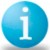John J. Hickey

Process capability (Cp) studies are usually performed whenever:

1. Engineering tolerances are reviewed against the observed variability of the process and/or new equipment is evaluated,
2. The capability of a process to meet customer specifications needs to be determined or
3. Process changes and/or improvements need to be evaluated.

Our rapid pace and highly competitive industrial environments often require expeditious decisions/actions in the above areas and an automated statistical method which minimizes sampling during these studies would be highly desirable. The sequential test method for process capability decisions described below will often result in a 50 percent sampling saving when compared with the most powerful classical tests. It is also both computer user and spreadsheet software friendly.

### Applying Wald’s Sequential Test Method to Process Capability Decisions

A process is defined as capable when the determined statistical control limits are at least equal to or within the specification limits and deemed incapable whenever the control limits lie outside of the specification limits. The capability index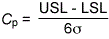where the upper specification limit (USL) and lower specification limit (LSL) represent the upper and lower specification limits and s the population standard deviation (STD), is a handy measure of process capability.

By convention, if Cp< 1, the process is deemed incapable; if Cp= 1 or Cp> 1, the process is considered marginally or definitively capable (e.g., Cp >= 1.33). Hence, for a selected USL-LSL specification range, any sampling decision on whether a process is capable is dependent upon the process standard deviations s0 (Cp> 1 ) s1 (Cp< 1 ) and corresponding Type I a and Type II b errors for hypothesis acceptance or rejection. With the exception of double sampling, most accept/reject inspection decisions assume that the observational numbers are independent of the results and require a predetermined sample size. Sequential test or inspection plan sample sizes are dependent on the outcome of the observations and require three decisions: 1) accept the test hypothesis, 2) take another observation and 3) reject the test hypothesis.

Historically, sequential testing results from the theory of sequential analysis that was created by WALD2 in 1943 for war time military equipment development and inspections. Numerous examples of the theory’s practical applications and sampling saving economies were later published1 by the Statistical Research Group of Columbia University. Examples of the mentioned 50 percent sampling economy can be found in the document. The basis for Wald’s sequential test are the probability inequality ratios: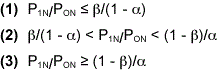where P0N, P1N are the probability density functions corresponding to the test H0 and alternate H1 hypothesis for the sequential set of observations X1…XN, and a, b are the selected Type I (producer’s risk) and Type II (consumer’s risk) errors. The test is continued when inequality ratio (2) applies and discontinued at the first occurrence of the ratios (1) or (3) resulting in the acceptance of hypotheses H0 or H1 respectively.

Handpicked Content:   Process Capability (Cp, Cpk) and Process Performance (Pp, Ppk) - What is the Difference?

As an example of test principle usage, consider the sequence of observations X1…XN from a normally distributed process with a known mean m and unknown standard deviation s, where the test hypothesis is s = s1. For each value of N, the probability ratio P1N/PON is calculated. Thus, the test hypothesis s = s1 when (3) P1N/PON >= (1-b)/a. The test is continued when (2) b/(1-a) < P1N/PON < (1-b)/a. The expansion of inequality (2) results in expression (4) below: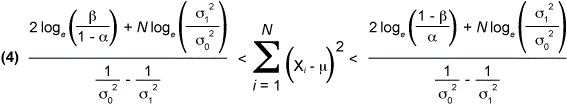and further simplification results in the straight line forms that are used to create the table (Table 2) and/or graph (Figure 1) of a Wald sequential test procedure for process variability:

Accept standard deviation s0 at step N if the summed observations * S (Xi – m)2 satisfy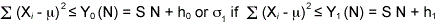and continue sampling if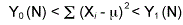where: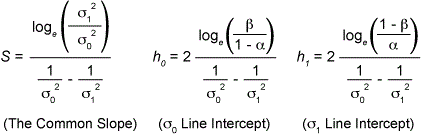*Note: In the cases where the mean m is unknown the sum of squares is replaced by the squares of the deviations from the sample mean and the limit N is replaced by N-1. Five useful points of both the operating characteristic Pa(s) and ASN curves can be calculated from the formulas in Table 1.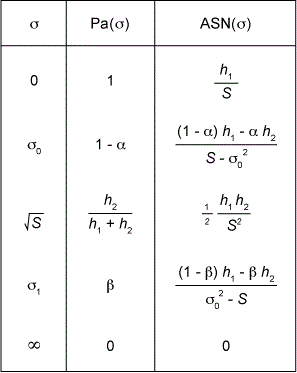Table 1: Five Useful Points

The ASN estimates the average number of samples required for a decision at a specified standard deviation s since the exact number is not known beforehand.

### A Process Capability Application Example1

A textile customer specifies an average burst strength and tolerance of 120 pounds +/- 50 pounds for each patch of manufactured cloth and further requires a minimum Cp of 1.33. The specification range (USL-LSL) in this case is 100 pounds and the maximum allowable process standard deviation calculates as 100/(6 x 1.33) = 12.5 pounds. The cloth manufacturer accepts the customer’s specification based on a standard deviation comparison of New Machine A with the historical results obtained for older equipment. The sequential sampling parameters s0=12.5, s1=24.3, a=0.001 and b=0.001 are chosen for the study. The Excel spreadsheet outputs are illustrated in Table 2, Figure 1 and Table 3. Table 3 lists an ASN of 23 for this test at the desired standard deviation level of 12.5. The test was terminated after 15 cumulative samples were taken. This is indicated by the asterisk in the immediate left column of the calculated accept STD1 (12.5) criteria sums in Table 2 and Figure 1 chart. The manufacturer has decided to produce the customer’s orders on New Machine A (Cp>= 1.33) since the older equipment is not capable.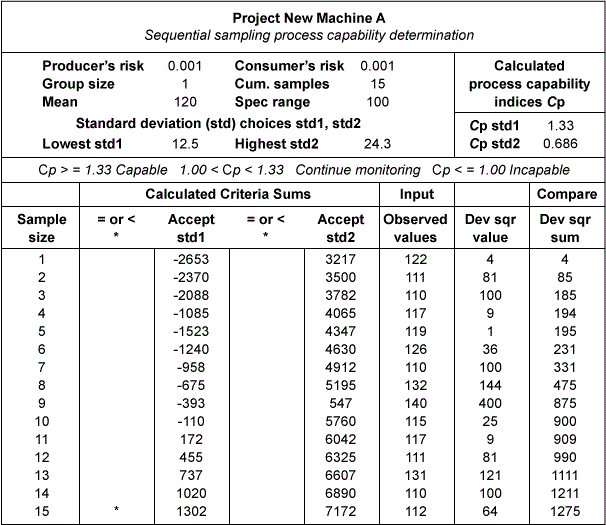Table 2: Sequential Sampling Process Capability Determination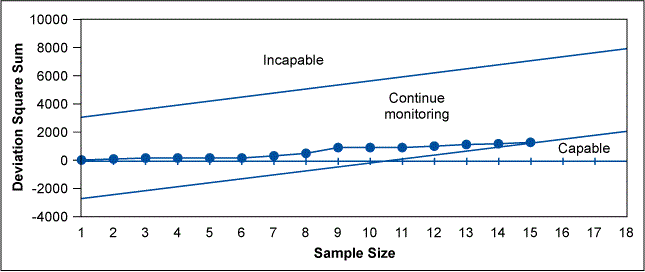Figure 1: Sequential Sampling Process Capability Plot, New Machine A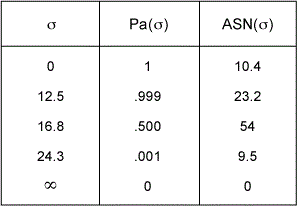Table 3: New Machine A

### Variance Sampling Economy

When a classical test of significance is applied to question of whether the sample variance s2 differs significantly from the desired population variance s0, a Chi-square test X2 is usually applied. The test hypothesis H0 specifies the population variance as H0: s2 = s02 and the alternate hypothesis H1: s12 > s02. If H0 is correct, then X2/f is the distribution for s2/s02 for f degrees of freedom. Thus, a rejection of the test hypothesis H0 occurs at some chosen significance level a such that

Handpicked Content:   Table of the Standard Normal (z) Distribution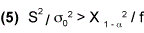The test’s sample size can be estimated from the power of the discriminating test3 needed to distinguish between the population variances s12 and s02 at f degrees of freedom: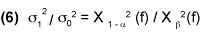The substitution of the textile process parameters, s0=12.5, s1=24.3, a=0.001, b=0.001, in the discriminating test equation (6) yields s12/s02=3.78=X.9992(f)/X.0012(f) at about 47 degrees of freedom, which translates into a required sample size of 48 for the classical test. The ASN required for a sequential test decision at a standard deviation level of s0=12.5 is 23 according to Table 3, resulting in an average sampling economy of 52 percent. For an unknown mean, the sample size is increased by one and the saving’s percentage is reduced to 50 percent.